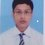# Jharkhand RMO 2015

This time RMO of jharkhand was more difficult than last year .. what do you all reckon what will be cut off for this year?..Note by Gyanendra Prakash
4 years, 10 months ago

This discussion board is a place to discuss our Daily Challenges and the math and science related to those challenges. Explanations are more than just a solution — they should explain the steps and thinking strategies that you used to obtain the solution. Comments should further the discussion of math and science.

When posting on Brilliant:

• Use the emojis to react to an explanation, whether you're congratulating a job well done , or just really confused .
• Ask specific questions about the challenge or the steps in somebody's explanation. Well-posed questions can add a lot to the discussion, but posting "I don't understand!" doesn't help anyone.
• Try to contribute something new to the discussion, whether it is an extension, generalization or other idea related to the challenge.

MarkdownAppears as
*italics* or _italics_ italics
**bold** or __bold__ bold
- bulleted- list
• bulleted
• list
1. numbered2. list
1. numbered
2. list
Note: you must add a full line of space before and after lists for them to show up correctly
paragraph 1paragraph 2

paragraph 1

paragraph 2

[example link](https://brilliant.org)example link
> This is a quote
This is a quote
    # I indented these lines
# 4 spaces, and now they show
# up as a code block.

print "hello world"
# I indented these lines
# 4 spaces, and now they show
# up as a code block.

print "hello world"
MathAppears as
Remember to wrap math in $$ ... $$ or $ ... $ to ensure proper formatting.
2 \times 3 $2 \times 3$
2^{34} $2^{34}$
a_{i-1} $a_{i-1}$
\frac{2}{3} $\frac{2}{3}$
\sqrt{2} $\sqrt{2}$
\sum_{i=1}^3 $\sum_{i=1}^3$
\sin \theta $\sin \theta$
\boxed{123} $\boxed{123}$

Sort by:

last time cut off was 45. by the way how much are you getting this time?

- 4 years, 10 months ago

If the roots of x^3+px^2+qx+r=0 are all real and +ve...prove pq-9r>=0 and q^3-27r^2>=0

- 4 years, 10 months ago

find all triplets such that (a,b,c) of positive integers such that a<=b<=c: 3 does not divide b.c and (1+1/a)(2+1/b)(3+1/c)=13

- 4 years, 10 months ago

In a group of seven persons every person is asked to write the sum of ages of all the other six persons.if all the seven sums form a six element set {63,64,65,66,68,70}..then find the individual ages assuming ages are whole numbers..

- 4 years, 10 months ago

one question was: prove that the roots of the equation x^3-3x^2-1 = 0 are never rational.

- 4 years, 10 months ago

By rational root theorem, the only possible rational roots of the above polynomial could be $\pm1$ which are not in this case. Therefore, the roots are Irrational of Imaginary.

Either it is not a RMO question or my approach is not complete.

- 4 years, 10 months ago

this is surely from RMO (jharkhand RMO 2015)...but i have solved this problem integrating 3 concepts : theory of equations, inequalities and then final proof by contradiction

- 4 years, 10 months ago

well i approached like this:- let the roots be a,b,c a+b+c=3 ab+bc+ac=0 abc=1 assuming roots to be rational..i took a=p1/q1,b as p2/q2 and c as p3/q3

so i got p1/q1+p2/q2+p3/q3=3---------eq.1 p1p2/q1q2 + p2p3/q2/q3 + p1p3/q1q3 = 0-----eq.2 and p1p2p3=q1q2q3 -----------eq-3

procceding with equation 1

i got after expanding and replacing q1q2q3 by p1p2p3...

recoiprocal of eq.2=3 (after three steps of monotonous algebraic expansion)

taking eq2 as x+y+z = 0 and then its reciprocal from above as 1/x+x/y+1/z = 3

by A.M-G.M we know that (x+y+z)(1/x+1/y+1/z)>=9

but here we are getting it as 3*0=0

therfore by contradiction roots can't be rational...

i suppose it's corrcet..

- 4 years, 10 months ago

so does my solution deserve any credit?

- 4 years, 10 months ago

I don't know, but if it is a RMO Question it should be solved somewhat the way you did it. I can't actually understand your solution, should use LaTex and you left the most part of your solution that is the steps with which you solved.

- 4 years, 10 months ago

ok..i will improve it

- 4 years, 10 months ago